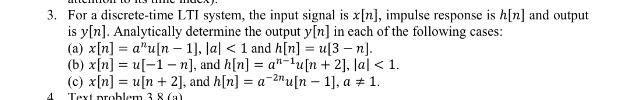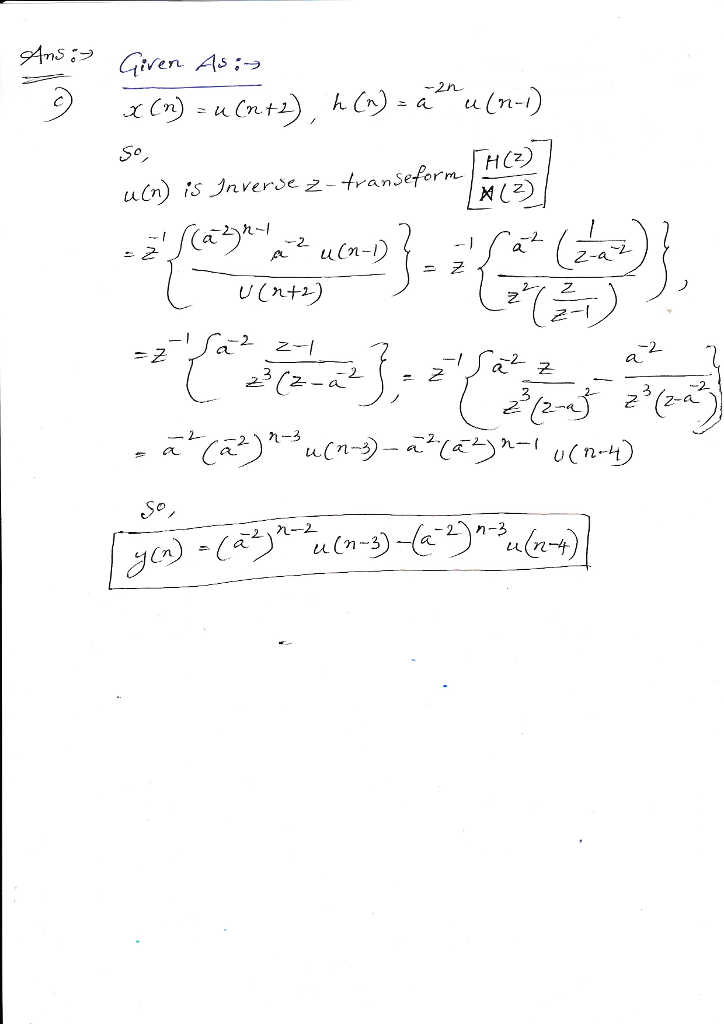# Homework Solution: For a discrete-time LTI system, the input signal is x[n], impulse response is h[n] and output is y[n]. Analyti…For a discrete-time LTI system, the input signal is x[n], impulse response is h[n] and output is y[n]. Analytically determine the output y[n] in each of the following cases: (a) x[n] = a^n u[n - 1], |a|